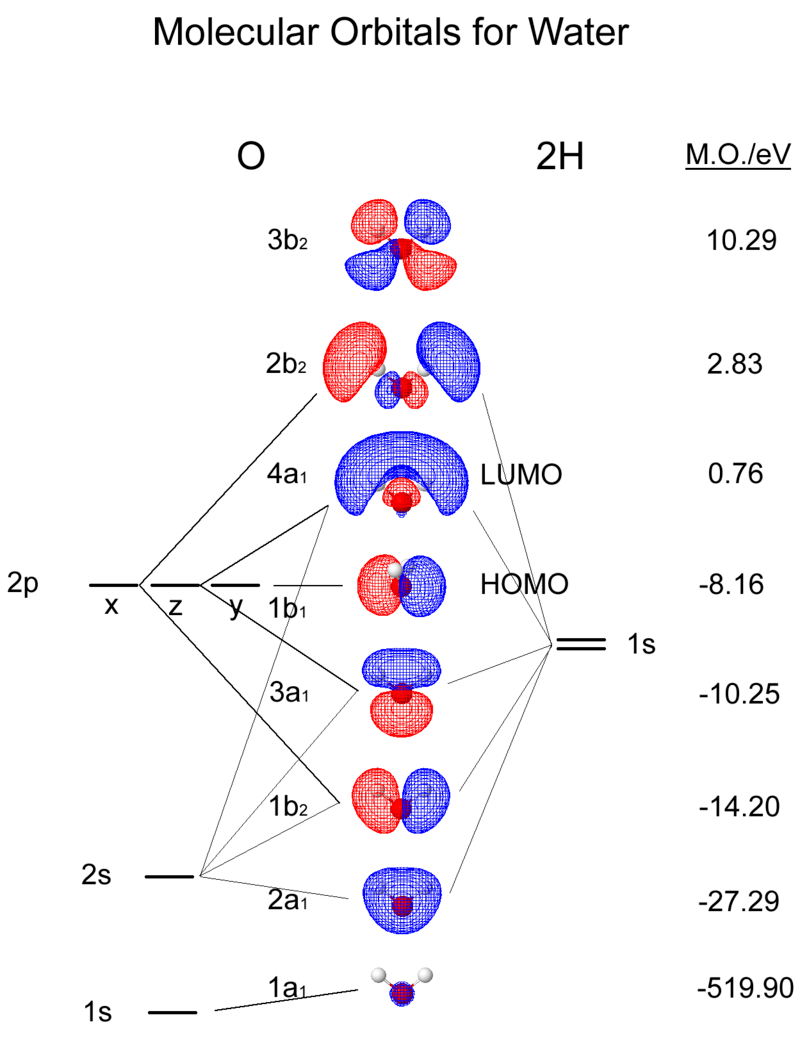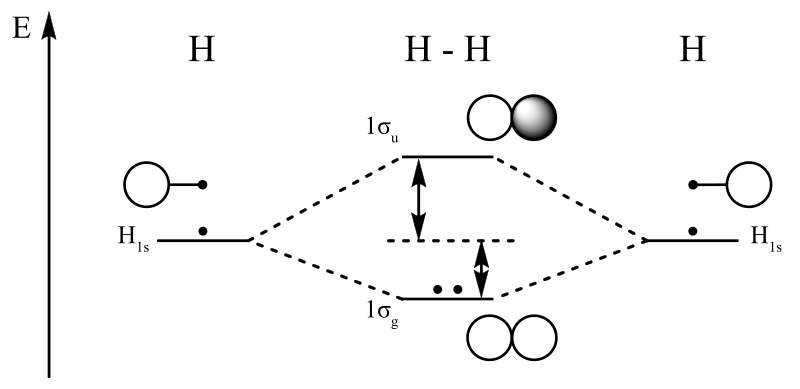# How to Calculate Bond Order from Molecular Orbital Diagram

Understanding the bond order of a molecule is a crucial step in analyzing its chemical properties and reactivity. The bond order, derived from a molecular orbital diagram, provides insights into the strength, stability, and nature of chemical bonds within a molecule. Before we delve into calculating bond order, it’s crucial to grasp the concept of molecular orbitals.

### Key Areas Covered

1. What are Molecular Orbitals
– Definition, Features
2. How to Calculate Bond Order from Molecular Orbital Diagram
– Formula, Steps, and Examples
3. How to Interpret Bond Order
– General Guidelines
4. FAQ: Calculating Bond Order from Molecular Orbital Diagram

### Key Terms

Bond Order, Molecular Orbital Diagram## What are Molecular Orbitals

Molecular orbitals are regions of space where electrons are likely to be found in a molecule. They are formed by the overlapping of atomic orbitals, which are regions of space where electrons are localized around individual atoms.

Molecular orbitals result from the linear combination of atomic orbitals, where atomic orbitals from different atoms overlap and create bonding and antibonding orbitals. Bonding orbitals are lower in energy and stabilize the molecule, while antibonding orbitals are higher in energy and destabilize the molecule. The bond order can be determined by comparing the number of electrons in bonding and antibonding orbitals.Figure 1: Molecular Orbital Diagram for Water

## How to Calculate Bond Order from Molecular Orbital Diagram

Bond order is calculated using the formula:

Bond Order (BO) = (Number of Electrons in Bonding Molecular Orbitals – Number of Electrons in Antibonding Molecular Orbitals) / 2

Here’s a step-by-step guide to calculating bond order from a molecular orbital diagram:

1. Draw the Molecular Orbital Diagram: Start by drawing the molecular orbital diagram for the molecule of interest. Molecular orbital diagrams show the distribution of molecular orbitals, including bonding and antibonding orbitals, as well as the energy levels of these orbitals. The most commonly used molecular orbital diagrams are for diatomic molecules (two atoms).
2. Determine the Number of Electrons: Next, you need to determine the number of electrons in the molecule. This information is generally provided in the question or can be deduced from the molecular formula of the compound. You’ll need to know the total number of valence electrons available.
3. Fill Electrons into the Molecular Orbitals: Following Hund’s Rule, you should start filling electrons into the molecular orbitals, beginning with the lowest-energy orbital and working your way up. Electrons are placed one by one in the molecular orbitals until you’ve accounted for all the electrons. It’s essential to follow the Pauli Exclusion Principle, which states that no two electrons in the same orbital can have the same spin.
4. Calculate the Bond Order: Once you’ve filled the electrons into the molecular orbitals, you can calculate the bond order using the formula mentioned earlier. Then, take the difference between the number of electrons in bonding molecular orbitals and antibonding molecular orbitals and divide it by 2. This will give you the bond order of the molecule.

### Examples of Calculating Bond Order from Molecular Orbital Diagram

Let’s look at some examples to illustrate how to calculate bond order from molecular orbital diagrams:

Hydrogen Molecule (H2): In the molecular orbital diagram for H2, both hydrogen atoms contribute one electron each to form a sigma (σ) bonding orbital. There are no antibonding orbitals in this case, so the bond order is calculated as follows:

Bond Order (BO) = (2 – 0) / 2 = 1

Oxygen Molecule (O2): In the molecular orbital diagram for O2, oxygen atoms contribute a total of 12 electrons. After filling the molecular orbitals, you’ll find 8 electrons in bonding orbitals and 4 electrons in antibonding orbitals. Therefore, the bond order is calculated as follows:

Bond Order (BO) = (8 – 4) / 2 = 2

## How to Interpret Bond Order

• If the bond order is 0, it indicates that there is no bond between the two atoms. In this case, the molecule is non-existent or unstable.
• A bond order of 1 represents a single bond, indicating a stable covalent bond between two atoms.
• A bond order of 2 represents a double bond, indicating a strong covalent bond where two pairs of electrons are shared between the atoms.
•  A bond order of 3 represents a triple bond, indicating an even stronger covalent bond where three pairs of electrons are shared between the atoms.
• Fractional bond orders between 0 and 1 typically indicate the presence of a resonance structure. In such cases, the actual bond is a hybrid of multiple resonance structures.
• Bond orders greater than 3 are possible in molecules with multiple bonds; for example, in some nitrogen oxides or certain transition metal compounds.

Furthermore, the bond order provides critical insights into a molecule’s properties:

• Stability: Higher bond orders indicate stronger and more stable bonds between atoms, making the molecule less reactive.
• Bond Length: Generally, higher bond orders result in shorter bond lengths because of the increased electron density between the nuclei.
• Bond Energy: Higher bond orders correspond to stronger covalent bonds with higher bond energies.Figure 2: Dihydrogen Molecular Orbital Diagram

## FAQ: Bond Order from Molecular Orbital Diagram

### Is bond order always positive?

• In molecular orbital theory, bond order is typically a non-negative value, as it represents the strength of the bond between two atoms. Bond order can also be zero, indicating the absence of a chemical bond, or it can be a positive integer or a fractional value, depending on the strength of the bond.

### How do you find the bond order of o2 in molecular orbital theory?

• To find the bond order of O2 in molecular orbital theory, subtract the number of anti-bonding electrons from the number of bonding electrons and then divide the result by 2.

### What is the bond order of the b2 molecular orbital diagram?

• The molecular orbital diagram for B2 (diboron) reveals a bond order of 1. This implies a single covalent bond between the two boron atoms. Moreover, the diagram shows that there are two bonding electrons and no antibonding electrons, resulting in a bond order of 1/2, which simplifies to 1.
##### Reference:

1. “Bond Order Formula.” Byjus’s.
2. “Bond Order and Lengths.” Libre Texts Chemistry.

##### Image Courtesy:

1. “Molecular Orbitals for Water”  By ChiralJon (CC BY 2.0) via Commons Wikimedia
2. “Dihydrogen-MO-Diagram” By CCoil (talk) – Own work (CC BY-SA 3.0) via Commons Wikimedia### About the Author: Hasini A

Hasini is a graduate of Applied Science with a strong background in forestry, environmental science, chemistry, and management science. She is an amateur photographer with a keen interest in exploring the wonders of nature and science.